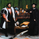# Forecasting - Holt’s Linear Trend Forecasting

1734 просмотров
Holt's Forecasting method

Holt (1957) extended simple exponential smoothing to allow the forecasting of data with a trend. This method involves a forecast equation and two smoothing equations ( one for the level and one for the trend):

Forecast equation: ŷ = l + h * b
Level equation: l = alpha * y + (1 - alpha) * (l + b)
Trend equation: b = beta * (l - l) + (1 - beta) * b

where:
l (or l) denotes an estimate of the level of the series at time t,
b (or b) denotes an estimate of the trend (slope) of the series at time t,
alpha is the smoothing parameter for the level, 0 ≤ alpha ≤ 1, and
beta is the smoothing parameter for the trend, 0 ≤ beta ≤ 1.

As with simple exponential smoothing, the level equation here shows that l is a weighted average of observation y and the one-step-ahead training forecast for time t, here given by l+b. The trend equation shows that b is a weighted average of the estimated trend at time t based on l-l and b, the previous estimate of the trend.

The forecast function is not flat but trending. The h-step-ahead forecast is equal to the last estimated level plus h times the last estimated trend value. Hence the forecasts are a linear function of h.
Информация о релизе: Minor fix in documentation.
Скрипт с открытым кодом

In true TradingView spirit, the author of this script has published it open-source, so traders can understand and verify it. Cheers to the author! You may use it for free, but reuse of this code in a publication is governed by House Rules. You can favorite it to use it on a chart.

Хотите использовать этот скрипт на графике?

## КомментарииUPD: use f instead of forecast The line forecast = l + b may be removed.
ОтветитьRafaelZioni
@RafaelZioni, thx for you suggestion, but I left it as such deliberately as a short-term forecast.
Anyway, you can update in the Inputes section:
ex = input(true, "Extend Right")
And then in the Main section:
fl := line.new(time, close, time + 60 * 60 * 24 * 6, f,
xloc.bar_time, extend=ex ? extend.right : extend.none, style=line.style_solid, color=color.blue, width=2)
Ответить
Профиль Настройка профиля Счёт и оплата Ваши друзья Монеты Мои запросы в поддержку Справочный центр Личные сообщения Чат Выйти